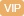| APP | 校园号 | 客服 客服热线：400-863-9889

400-863-9889（1）30=2×3×□
70=2×□×□
45=□×□×□
105=□×□×□
（2）12×20=10×□
24×25=100×□
15×16=□×20
15×18=□×□
12×□=18×□
□×□=42×□
【考点】合数分解质因数口算与笔算乘法-两位数乘两位数【专题】数的整除；运算顺序及法则．
【分析】分解质因数就是把一个合写成几个质的连形式，一般先从简单的质试解；由此解决问题．

【解答】解：30=×3×5
24=42×2
2×20=22×0=10×24
2×5=64×25=100×6
702×5×7
05=×5×7
15×13×5×4=12×20
1218=8×12

【点评】此题考查数分解质因数的法以及运定律的灵运，是基础题型．

0/0